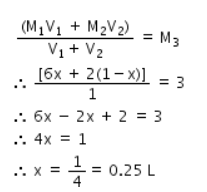# In preparing solution of a given concentration from solution of varying

In preparing solution of a given concentration from solution of varying concentration, the following formula is used i.e M1V1+M2V2=M3V3.what volume of 6M HCl and 2M HCl should be mixed to prepare 1000ml of 3M HCl?

Let us suppose that the volume of 6 M HCl (V1) = x L
Therefore, the volume of 2 M HCl required to make 1 L of 3 M HCl (V2) = (1- x) L
Let us take M1 = 6M and M2 = 2M,
As given, we know that, Molarity of a mixture is given by the equation:Therefore, Volume of 6M HCl = 0.25L
and Volume of 2 M HCl = 1-0.25 = 0.75 L